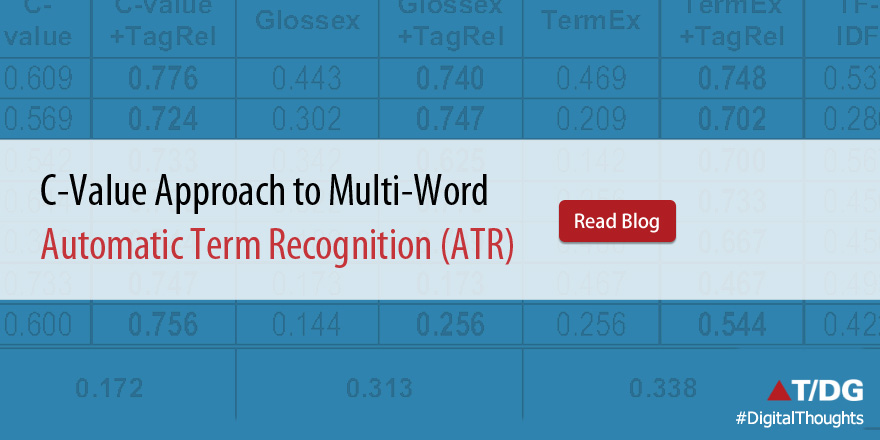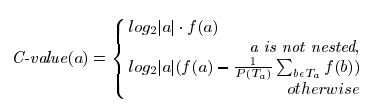# C-value approach to multi-word automatic term recognition (ATR)C-value is a domain-independent method for multi-word ATR which aims to increase the extraction of nested terms. It aims to get more accurate terms, especially those nested terms, such as ”MUTUAL INFORMATION” nested in longer term ”MUTUAL INFORMATION FEATURE EXTRACTION CRITERIA”. The C-value approach combines linguistic and statistical information.

• The linguistic information consists of the part-of-speech tagging of the corpus, the linguistic filter constraining the type of terms extracted, and the stoplist
• The statistical part combines statistical features of the candidate string, in a form of measure that is also called C-value, emphasis being placed on the statistical part.
The statistical part
The C-value statistical measure assigns a termhood to a candidate string, ranking it in the output list of candidate terms. The measure is built using statistical characteristics of the candidate string.

1. The total frequency of occurrence of the candidate string in the corpus.
2. The frequency of the candidate string as part of other longer candidate terms.
3. The number of these longer candidate terms.
4. The length of the candidate string (in number of words).

The measure of termhood, called C-value is given asWhere

a is the candidate string,

f (.) is its frequency of occurrence in the corpus,

Ta is the set of extracted candidate terms that contain a,
P(Ta) is the number of these candidate terms.

The algorithm
Step 1: The corpus is tagged, since we need to filter the desired type of terms.
Step 2: We extract those terms which satisfy the linguistic filters and frequency
threshold
Step 3: We calculate the C-value for each of the candidate term at this stage. Since we read input text sentence by sentence, we can extract terms in the input sentence, record the frequency of occurrence, count t(a) (the number of being nested) and c(a) (the number of longer terms), and then store them in memory for later use.

Calculation of c(a) and t(a)

• A candidate term whose length is bigger than 2 may contain several shorter terms.
• For example term “Artificial Neural Network Classifier” may contain five shorter candidate terms “Artificial Neural Network”, “Neural NetworkClassifier”, “Artificial Neural”, “Neural Network”, ”Network Classifier”.
• For computational efficiency, we should cumulate the frequency of c(a) and t(a).
• In this example for candidate term Artificial Neural Network”, c(a) increases by 1, t(a) increase by 1.
• For candidate term Artificial Neural Network Classifier”, c(a)=0, t(a)=0, because it is not nested.
• For candidate term Artificial Neural”, c(a) increases by 2, t(a) increases by 2, because it is nested by two longer terms Artificial Neural Network” and Artificial Neural Network Classifier”.
• Once we complete the input, we can calculate the C-value for each candidate term since we have recorded all the parameters needed to calculate the C-value.

Example

• To show how C-value method works.
• We can find candidate terms POSSIBILITY DENSITY FUNCTION and POSSIBILITY DENSITY in the corpus.
• f(POSSIBILITY DENSITY FUNCTION) = 225, f(POSSIBILITY DENSITY) =257. c(POSSIBILITY DENSITY FUNCTION)=0, so C-value for POSSIBILITY DENSITY FUNCTION is calculated by C-value = log2 |a| * f(a) = log2 3 * 225 = 358, c(POSSIBILITY DENSITY) = 1, t(POSSIBILITY DENSITY)=225. So C-value for POSSIBILITY DENSITY is calculated by C-value = log2|a|(f(a) -t(a)/c(a))=log2(2)(358-225/1)=133.